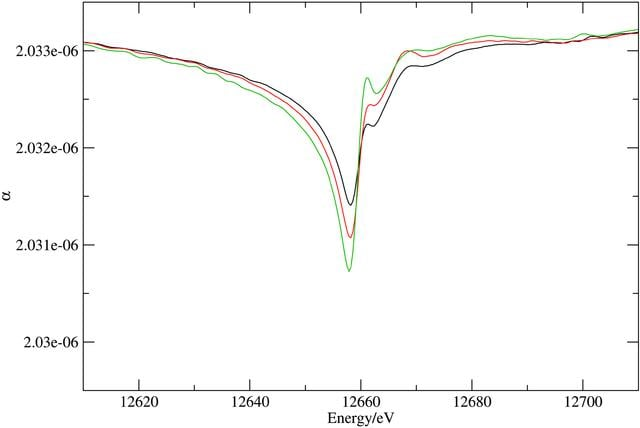disable zoom     view article Figure 8 Eigenvalues of the refraction tensor at the Se K edge. The plot represents the spectra of the three eigenvalues of the α tensor as a function of X-ray energy at the Se K edge. The red curve represents the eigenvalues corresponding to the y direction (i.e. the crystal b axis). The other two curves represent eigenvalues corresponding to two mutually orthogonal directions in the xz plane which vary with X-ray energy [i.e. there is some orientational dispersion (Lippmann et al., 1998) in this plane].JOURNAL OFAPPLIEDCRYSTALLOGRAPHY
ISSN: 1600-5767
Volume 38| Part 1| January 2005| Pages 168-182